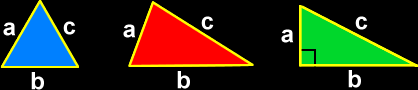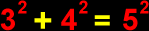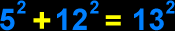# The Geometry of Triangles

Definitions and formulas for the area of a triangle, the sum of the angles of a triangle, the Pythagorean theorem, Pythagorean triples and special triangles (the 30-60-90 triangle and the 45-45-90 triangle)
Just scroll down or click on what you want and I'll scroll down for you!

 examples of triangles area of a triangle sum of the anglesof a triangle The Pythagorean Theorem special Pythagoreantriples special triangles45-45-90 & 36-60-90

 Examples of triangles:The area of a triangle:The sum of the angles of a triangle:Always, always, ALWAYS!The Pythagorean Theorem:This formula is for right triangles only!The sides, a and b, of a right triangle are called the legs, and the side that is opposite to the right (90 degree) angle, c, is called the hypotenuse.  This formula will help you find the length of either a, b or c, if you are given the lengths of the other two.Some special Pythagorean numbers: These are called Pythagorean triples.The reason that they are so special is that they are whole numbers -- none of those weird decimals, fractions or radicals!